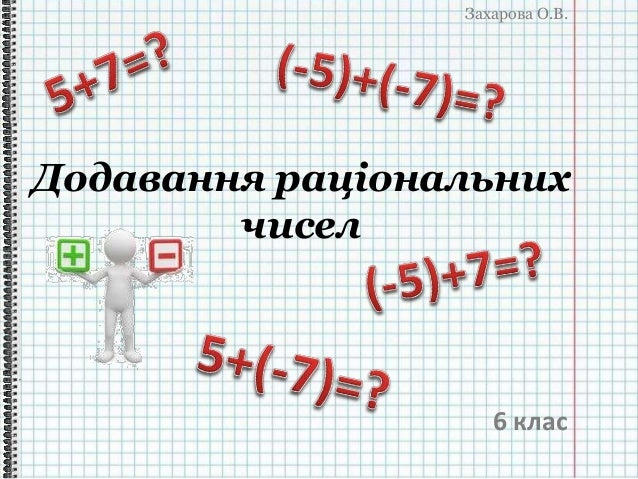Successfully reported this slideshow.Upcoming SlideShare
×

# Додавання раціональних чисел

1,398 views

Published on

Презентація для 6-го класу з курсу математики: "Додавання раціональних чисел"

Published in: Education
• Full Name
Comment goes here.

Are you sure you want to Yes No• Be the first to comment

### Додавання раціональних чисел

1. 1. Захарова О.В. Додавання раціональних чисел 6 клас
2. 2. Захарова О.В. Модуль Знак Раціональне число Раціональне число Модуль Знак 160 160 + - 89,5 89,5 + -9,4 9,4 - 5 3 7 5 3 7
3. 3. Захарова О.В. З’ясуємо а b с Модуль ? Знак ? c a і b додатні а – додатне, b – від’ємне а – від’ємне, b - додатне a і b від’ємні
4. 4. Захарова О.В. a і b додатні Сума двох додатних чисел є числом додатним 0 1 5 12 +7
5. 5. Захарова О.В. a і b додатні Сума двох додатних чисел є числом додатним 0 1 5 12 +7 1.|5|=5; |7|=7 2.5+7=12 3.12 5 7
6. 6. Захарова О.В. а – додатне, b – від'ємне 0 1 5 +(-7) -2
7. 7. Захарова О.В. а – додатне, b – від'ємне 0 1 5 +(-7) -2 1.|5|=5; |-7|=7 (|-7|>|5|) 2.7-5=2 3.-2 5 7
8. 8. Захарова О.В. а – від’ємне, b – додатне 0 1-5 +7 2
9. 9. Захарова О.В. а – від’ємне, b – додатне 0 1-5 +7 2 1.|-5|=5; |7|=7 (|7|>|-5|) 2.7-5=2 3.2 5 7
10. 10. Захарова О.В. a і b від’ємні 0 1-5 +(-7) -12
11. 11. Захарова О.В. a і b від’ємні 0 1-5 +(-7) -12 1.|-5|=5; |-7|=7 2.5+7=12 3.-12 57
12. 12. Захарова О.В. Проаналізуємо приклад числа за знаком дія порівняння модулів знак суми 5+7=12 однакові додатні + + 5+(-7)=-2 різні - |5|<|-7| більше від'ємне - -5+7=2 різні - |-5|<|7| більше додатне + -5+(-7)=-12 однакові від’ємні + -
13. 13. Захарова О.В. ПРАВИЛО додавання раціональних чисел з однаковими знаками 1.Знайти модулі доданків 2.Додати модулі доданків 3.Перед отриманим числом поставити знак доданків
14. 14. Захарова О.В. ПРАВИЛО додавання раціональних чисел з різними знаками 1.Знайти модулі доданків 2.Від більшого модуля відняти менший модуль 3. Перед отриманим числом поставити знак того з доданків, модуль якого більший
15. 15. Захарова О.В. -5+5=|-5|-|5|=5-5=0; 0 1-5 +5 0 1 5 +(-5) 5+(-5)=|5|-|-5|=5-5=0; a і b протилежні числа Сума протилежних чисел дорівнює 0
16. 16. Захарова О.В. Як будемо записувати в зошиті 1. 13,2+7,09=20,29 2. -11,5+(-8,05)=-(11,5+8,05)=-19,55 3. 5,4+(-8,9)=-(8,9-5,4)=-4,5 4. -3,1+7,06=+(7,06-3,1)=7,06-3,1=3,96 Знайти суму:
17. 17. Захарова О.В. Закони додавання Переставний закон додавання: Сполучний закон додавання:
18. 18. Захарова О.В. Застосуємо на практиці Знайти суму: -2,8+3,2+(-1,2)+5,8 Спосіб 1. Групуємо за однаковими знаками -2,8+3,2+(-1,2)+5,8= -2,8+(-1,2)+3,2+5,8= =-(2,8+1,2)+(3,2+5,8)=-4+9=9-4=5 Спосіб 2. Групуємо з різними знаками -2,8+3,2+(-1,2)+5,8= = (-2,8+5,8)+(3,2+(-1,2))= = (5,8-2,8)+(3,2-1,2)= 3+2=5
19. 19. Захарова О.В. • Сума майна і майна є майном • Сума боргу і боргу є боргом • Сума майна і боргу дорівнює їх різниці • Сума майна і такого самого боргу дорівнює нулю Брахмагупта (VIIст.) – індійський математик